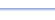Program: SYNTHDIV

SYNTHDIV

• Divides a 3rd degree polynomial by a linear binomial.

Useful for:

• SAT
• ACT
• Algebra 1
• Algebra 2
• Precalculus
• Calculus

See full list of programs

Example

Q: Divide:

Solution:

Run SYNTHDIV.  How?

Enter the coefficients
of the polynomial:
A = 1, B = 2,
C = -1, D = -2

Enter E = 3 because
the divisor is x-3

The program reports
the coefficients of the
polynomial and the
remainder.

Interpretation:or